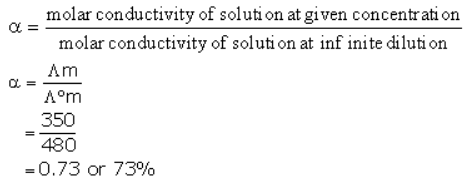Courses

# Test: Kohlrausch’S Law

## 10 Questions MCQ Test Chemistry Class 12 | Test: Kohlrausch’S Law

Description
This mock test of Test: Kohlrausch’S Law for Class 12 helps you for every Class 12 entrance exam. This contains 10 Multiple Choice Questions for Class 12 Test: Kohlrausch’S Law (mcq) to study with solutions a complete question bank. The solved questions answers in this Test: Kohlrausch’S Law quiz give you a good mix of easy questions and tough questions. Class 12 students definitely take this Test: Kohlrausch’S Law exercise for a better result in the exam. You can find other Test: Kohlrausch’S Law extra questions, long questions & short questions for Class 12 on EduRev as well by searching above.
QUESTION: 1

Solution:
QUESTION: 2

Solution:
QUESTION: 3

### Which of the following statements is correct?

Solution:

The correct answer is Option D.

The molar conductivity increases with decrease in concentration both, for weak and strong electrolytes.

This is because the total volume, V, of a solution containing one mole of electrolyte also increases. It has been found that a decrease in k on dilution of a solution is more than compensated by an increase in its volume.

QUESTION: 4

Molar conductivity for a compound AB is 145.0 Scm2mol-1 and for CB is 110.1 Scm2mol-1. Limiting molar conductivity for A+ is 73.5 Scm2mol-1. What is limiting molar conductivity for C+?

Solution:
QUESTION: 5

Kohlrausch’s Law shows that:

Solution:
QUESTION: 6

Conductivity of a solution contained within 1 centimetre cube volume is known as:

Solution:
QUESTION: 7

Kohlrausch’s Law is applicable:

Solution:
QUESTION: 8

At 300K molar conductivity of solution A is 350 units, and at infinite dilution the molar conductivity of the same sample is 480 unit. Predict the percentage dissociation of the electrolyte.

Solution:QUESTION: 9

For weak electrolytes ∧mo cannot to be calculated by extrapolation of ∧mo vs (c)1/2graph. Which of the following is not the reason?

Solution:
QUESTION: 10

With increase in temperature the degree of dissociation of electrolyte:

Solution: